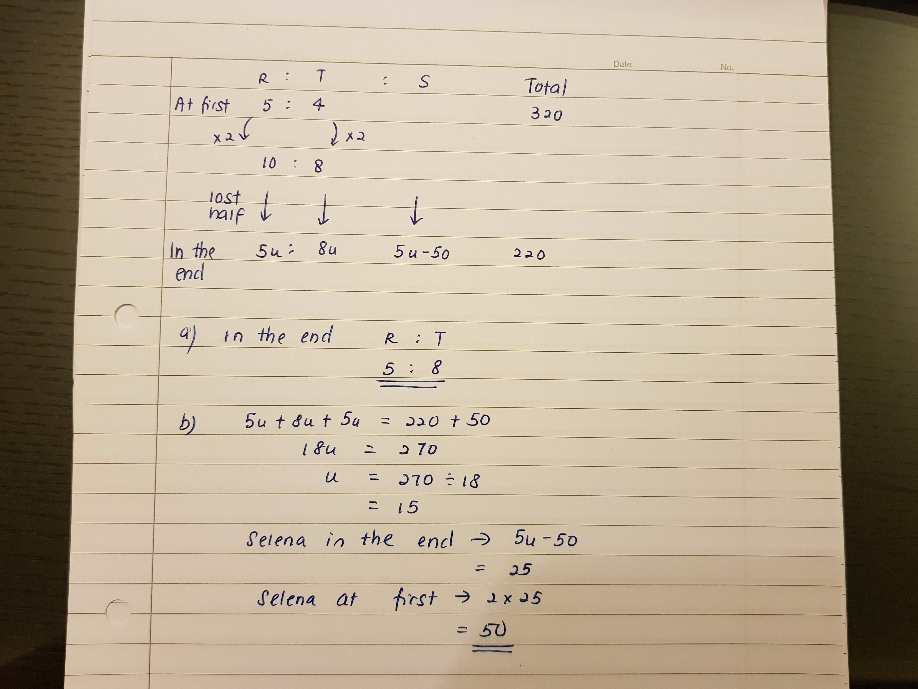# Question

At a game, Selena, Rihanna and Tina had a total of 320 clips. Selena had the least number of clips. The ratio of Rihanna’s clips to Tina’s clips was 5:4 at first. After Selena and Rihanna had each lost 1/2 of their clips, Rihanna had 50 clips more than Selena. The girls had a total of 220 clips in the end.

a) What is the ratio of the number of Rihanna’s clips to the number of Tina’s clips at the end of the game?

b) How many clips did Selena have at first?

 Rihanna : Tina : Selena At first 5 units : 4 units : Selena Cards lost 1/2 x 5 units = 2.5 units Unchanged 1/2 Selena Cards left in the end 5 units – 2.5 units = 2.5 units : 4 units : Selena – 1/2 Selena = 1/2 Selena OR 2.5 units – 50

 (a)   Selena’s cards left in the end → 2.5 units – 50   Cards left in the end = 2.5 units + 4 units + 2.5 units – 50 = 9 units – 50   9 units – 50 = 220   9 units = 220 + 50              = 270   1 unit = 270 ÷9            = 30   Rihanna’s cards left in the end = 2.5 units = 2.5 x 30 = 75   Tina’s cards left in the end = 4 units  = 4 x 30  = 120   Rihanna’s cards : Tina’s cards 75 : 120 5 : 8 (Ans) (b)   Selena’s cards left in the end → 2.5 units – 50  = (2.5 x 30) – 50  = 75 – 50  = 25   Since she lost half of her cards, her number of cards at first should be twice of what was left in the end.   Selena’s cards at first = 25 x 2                                         = 50 (Ans)

1 Reply 0 Likes

Hi may I know which part of the English says half of Selena is 2.5u?

1 Reply 0 Likes

Hi I understand the part where half of Selena less off 50 but can’t grasp why half of Selena is 2.5(u). I was try to understand the English but to not avail.

1 Reply 0 Likes

Noted thanks

0 Replies 0 Likes

 Rihanna : Tina : Selena At first 5 units : 4 units : Selena Cards lost x 5 units = 2.5 units Unchanged –  1/2 Serene Cards left in the end 5 units – 2.5 units = 2.5 units : 4 units : Serene at first – 1/2 Serene = 1/2 Serene OR 2.5 units – 50

 (a)   Selena’s cards left in the end → 2.5 units – 50   Cards left in the end = 2.5 units + 4 units + 2.5 units – 50 = 9 units – 50   9 units – 50 = 220   9 units = 220 + 50               = 270   1 unit = 270 ÷9            = 30   Rihanna’s cards left in the end = 2.5 units = 2.5 x 30 = 75   Tina’s cards left in the end = 4 units = 4 x 30 = 120   Rihanna’s cards : Tina’s cards 75 : 120 5 : 8 (Ans) (b) Selena’s cards left in the end → 2.5 units – 50  = (2.5 x 30) – 50  = 75 – 50  = 25   Since she lost half of her cards, her number of cards at first should be twice of what was left in the end.   Selena’s cards at first = 25 x 2                                            = 50 (Ans)

0 Replies 0 Likes0 Replies 0 Likes